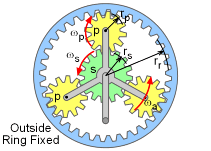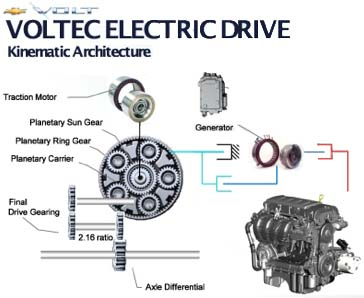Ch 5. Rigid Body General Motion Multimedia Engineering Dynamics Fixed Axis Rotation Plane Motion Velocities Zero Velocity Point Plane Motion Accelerations Multiple Gears Rot. Coord. Velocities Rot. Coord. Acceleration
 Chapter - Particle - 1. General Motion 2. Force & Accel. 3. Energy 4. Momentum - Rigid Body - 5. General Motion 6. Force & Accel. 7. Energy 8. Momentum 9. 3-D Motion 10. Vibrations Appendix Basic Math Units Basic Equations Sections Search eBooks Dynamics Fluids Math Mechanics Statics Thermodynamics Author(s): Kurt Gramoll ©Kurt GramollSTATICS - CASE STUDY IntroductionProblem Diagram (s = sun gear, p = planet gear, a = arm, planet gear connector) Cooks at Let Us Dine-R are not happy with the current salad spinning machine. It is slow and always breaks. One suggestion is to design a high-tech salad spinner using an epicyclical gear mechanism. What is known: The outside ring gear radius, rr, is set at 2.5 in and does not rotate (fixed). The handle spin rate, wa, is 1 rev/s. The sun gear is rigidly attached to the inside salad bowl container but is not fixed to the handle. The possible gear choices for the sun and planet gears are 0.5 in, 0.75 in, 1.0 in, and 1.5 in. Question Using an epicyclical gear mechanism, what gear radii (rs and rp) are best to produce a high spin rate of the container bowl (i.e. sun gear, ωs) with a moderate spin rate of the handle (ωa)? Approach Use the equation for motion around a fixed point. The tangent velocities of two touching gears are equal. The handle angular velocity will be directly related to the planet gear, which will in turn be related to the sun gear.Planetary Gear System in the Volt Electric Car

Practice Homework and Test problems now available in the 'Eng Dynamics' mobile app
Includes over 400 problems with complete detailed solutions.
Available now at the Google Play Store and Apple App Store.